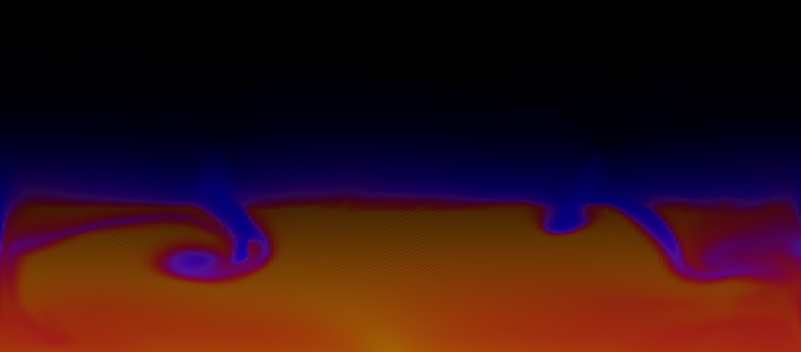﻿ Atmosphere ﻿@Template

# AtmospheresThis is very much work in progress, but here's a simple simulation of a 2-dimensional atmosphere.

Please don't click the following button, because it'll cause environmental damage:
The colour shows the temperature of the gas if it was adiabatically compressed to 1 atmosphere.
There's heating at the ground level, and cooling where it's high enough for the density to be below a threshold.
There are conserved quantities in each grid cell:
• mass
• momentum in the x direction
• momentum in the y direction
• The temperature of the air, if it was adiabatically compressed to 1 atmosphere, multiplied by the mass of the air. In real life, I'm not totally sure whether this would be conserved - I could check, but I haven't yet. It may not be.
These 4 conserved quantities make up the state of the system.
The timestep works like this:
For each edge between cells, work out a speed normal to the edge. So the left hand side of each square cell has a horizontal speed. The bottom of each square cell has a vertical speed. This is just an average of the 2 cells separated by the edge. You also need to add something proportional to the pressure difference across the edge, otherwise you get a numerical artifact.
The advection step works like this:
For each cell, we have 4 routes out: the 4 edges. The total that would leave is just the sum of the speeds * dt (speeds are in units of grid cell per second). This gets limited, so that the sum ends up being less than a certain fraction of the total contents of the grid cell.
Then each of the conserved quantities has a fraction moved across the edge, proportional to this limited speed.
Next is to update px and py with the pressure gradient and gravity. The pressure gradient is calculated from the square to the left to the square to the right of the current square. And again for vertical.
Then an extremely approximate equation of state is used to recalculate the pressures in each cell, based on the mass and the temperature-at-one-bar.
In terms of physical accuracy, I haven't yet made the equation of state accurate, nor have I checked that mass * temperature if adiabatically compressed to 1 atm, is conserved. I'd also like to add moisture content, and add clouds.
To visualise, I'm using Javascript's imageData stuff: it gives you access to the individual pixels. The complication is how opaque javascripts low level stuff is. If you set the pixels to a number, say 100, it has to guess whether that number is an integer or a byte, and if it gets it wrong, there's a huge performance penalty.